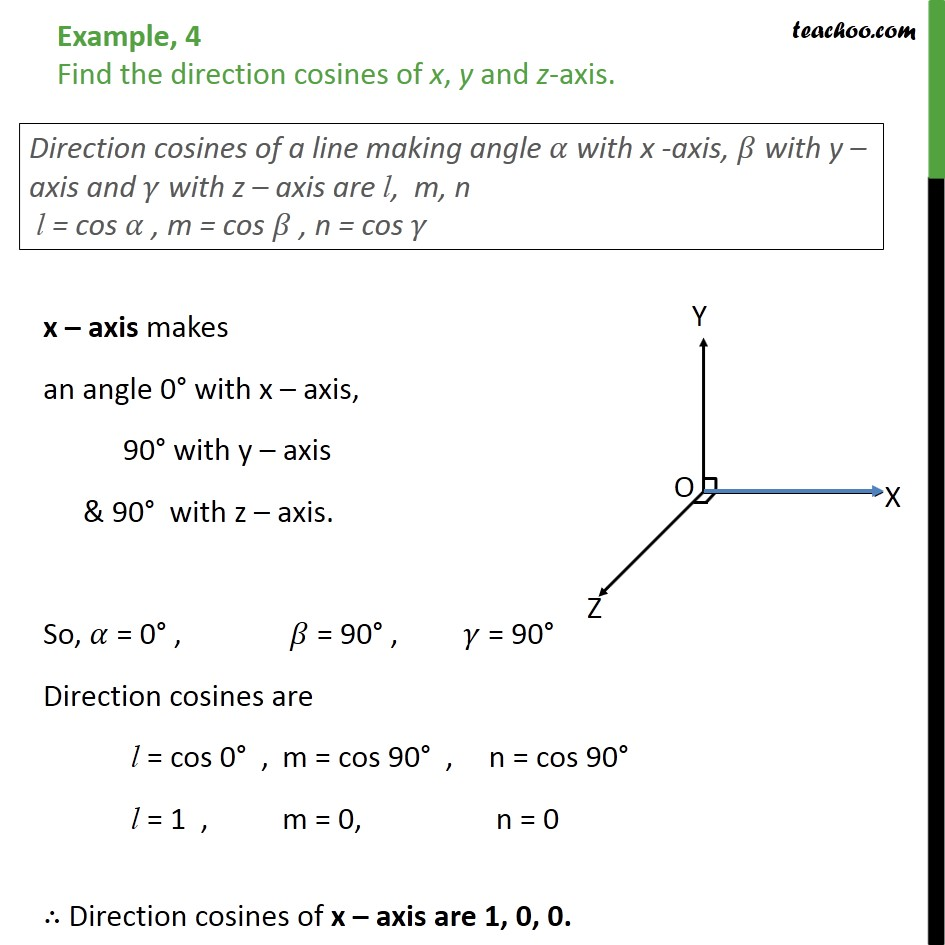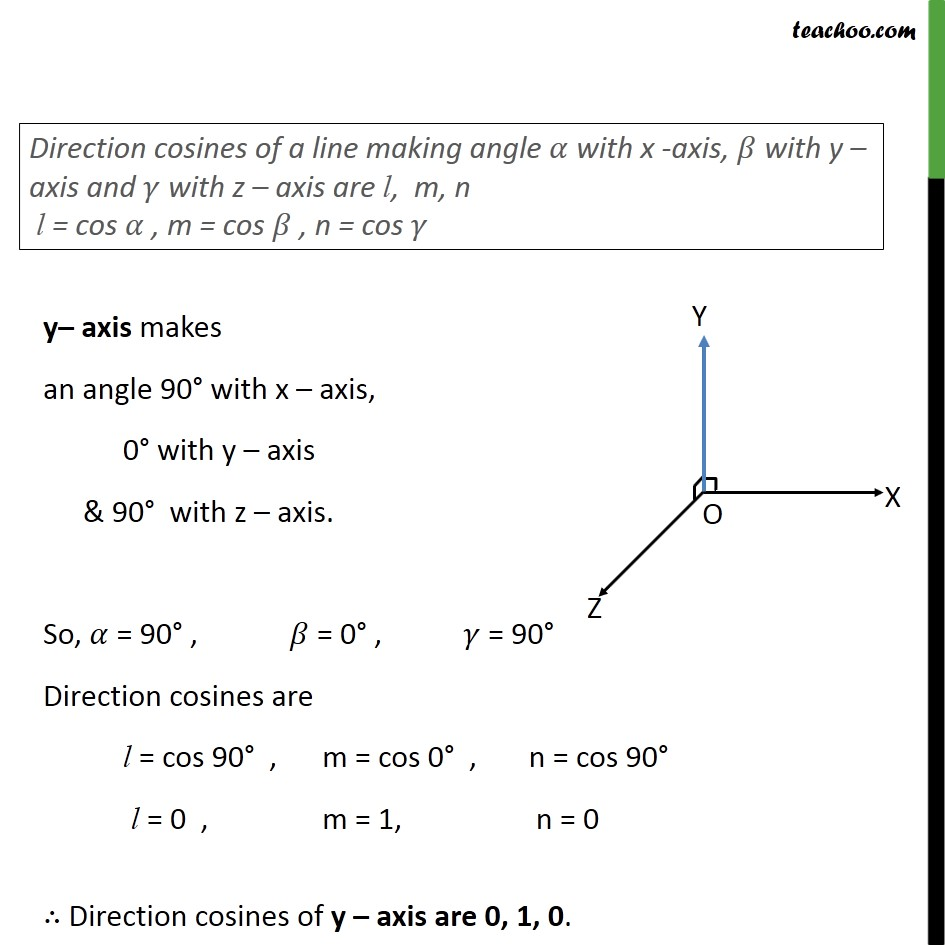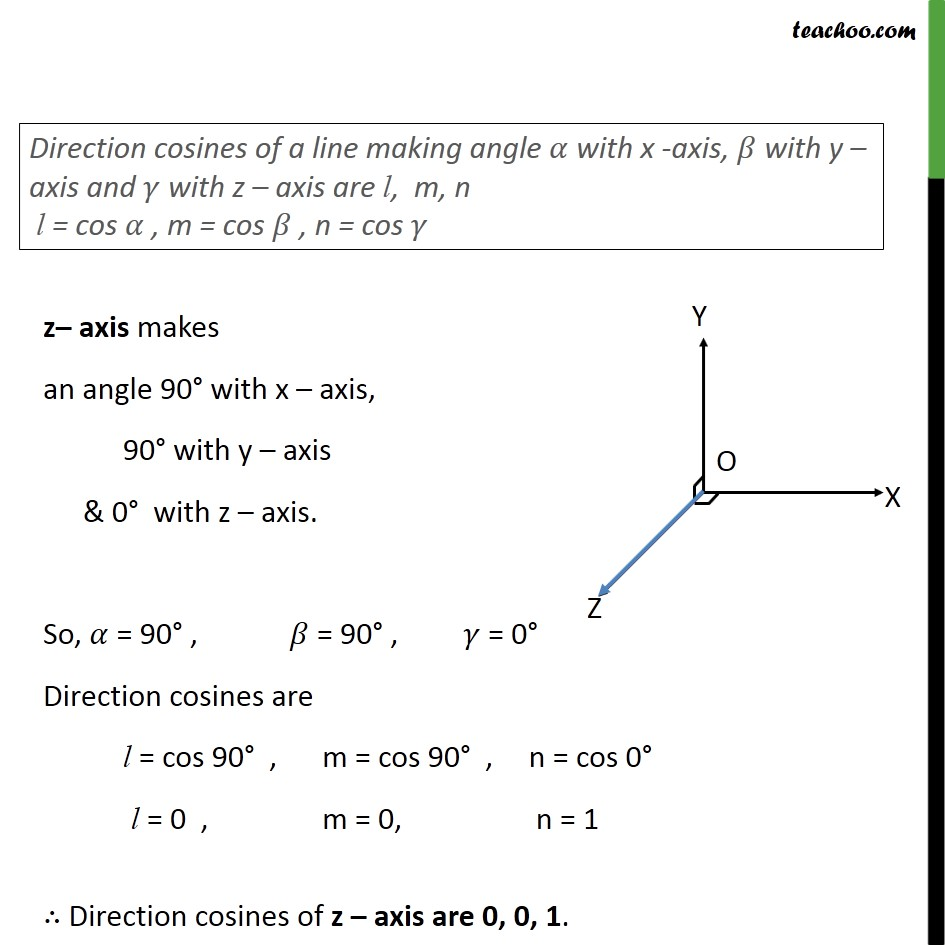1. Chapter 11 Class 12 Three Dimensional Geometry
2. Concept wise
3. Direction cosines and ratios

Transcript

Example, 4 Find the direction cosines of x, y and z-axis. x axis makes an angle 0 with x axis, 90 with y axis & 90 with z axis. So, = 0 , = 90 , = 90 Direction cosines are l = cos 0 , m = cos 90 , n = cos 90 l = 1 , m = 0, n = 0 Direction cosines of x axis are 1, 0, 0. y axis makes an angle 90 with x axis, 0 with y axis & 90 with z axis. So, = 90 , = 0 , = 90 Direction cosines are l = cos 90 , m = cos 0 , n = cos 90 l = 0 , m = 1, n = 0 Direction cosines of y axis are 0, 1, 0. z axis makes an angle 90 with x axis, 90 with y axis & 0 with z axis. So, = 90 , = 90 , = 0 Direction cosines are l = cos 90 , m = cos 90 , n = cos 0 l = 0 , m = 0, n = 1 Direction cosines of z axis are 0, 0, 1.

Direction cosines and ratios